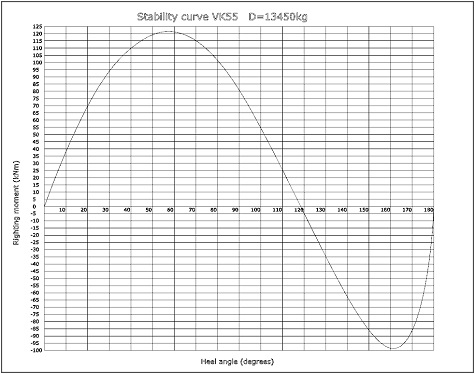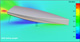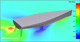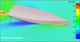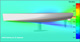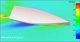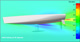Watch dynamic videos

Sails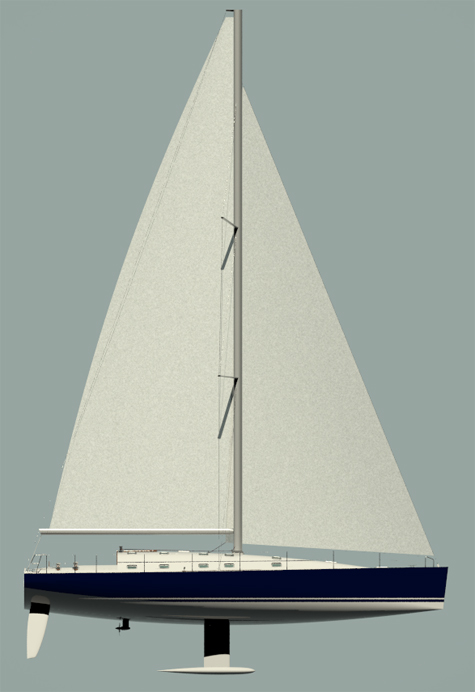VK55 Technical data Length overall = 16,8m Length waterline = 16,1m Beam overall = 4,8m Beam waterline = 3,5m Depth midsection = 4,3m Free board midsection = 1,7m Draft = 2,56m Displacement = 13450kg Ballast = 3720kg Engine VH4.80-59kW(80.3hp) Mainsail = 86m2 Genoa = 80m2 Spinnaker = 260m2 I = 18.8m J = 7.6m P = 20.2m E = 7.3m Windward balance point at RM30:    RM30 = 93,8kNm    Yaw = 5.4degrees    V_wind = 18.8knots    V_hull = 9.3knots Water = 340l Calorifier = 55l Fuel = 220l Gray&black = 120l Boat design category CE: A+ Certification: Ocean plus Design: VK Yacht

Stability curve